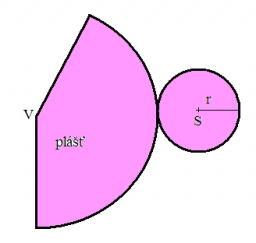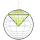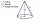# The volume

The volume of the cone is 94.2dm³, the radius of the base is 6 dm Calculate the surface of the cone.

S =  235.6103 dm2

### Step-by-step explanation:Did you find an error or inaccuracy? Feel free to write us. Thank you!Tips to related online calculators
Tip: Our volume units converter will help you with the conversion of volume units.
Pythagorean theorem is the base for the right triangle calculator.

#### You need to know the following knowledge to solve this word math problem:

We encourage you to watch this tutorial video on this math problem:

## Related math problems and questions:

• Rotating coneCalculate the volume and the surface area of a rotating cone of base radius r = 2.3 dm and a height h = 46 mm.
• The pyramid 4sThe pyramid with a rectangular base measuring 6 dm and 8 dm has a side edge of length 13 dm. Calculate the surface area and volume of this pyramid.
• Cone - from volume surface areaThe volume of the rotating cone is 1,018.87 dm3, and its height is 120 cm. What is the surface area of the cone?
• The rotatingThe rotating cone has a height of 0.9 m and the diameter of the base is 7.2 dm. Calculate the surface of the cone. (Hint: use Pythagorean theorem for a side of cone)
• The diagram 2The diagram shows a cone with slant height 10.5cm. If the curved surface area of the cone is 115.5 cm2. Calculate correct to 3 significant figures: *Base Radius *Height *Volume of the cone
• CalculateCalculate the cone's surface and volume that results from the rotation of the right triangle ABC with the squares 6 cm and 9 cm long around the shorter squeegee.
• PrismCalculate the height of the prism having a surface area 448.88 dm² wherein the base is square with a side of 6.2 dm. What will be its volume in hectoliters?
• The cone - S,VCalculate the volume and surface area of the cone if its radius r = 6 cm and side s = 10 cm.
• How toHow to find a total surface of a rectangular pyramid if each face is to be 8 dm high and the base is 10 dm by 6 dm.
• Surface of the coneCalculate the surface of the cone if its height is 8 cm and the volume is 301.44 cm3.
• Rotating coneFind the rotating cone's surface and volume if its side is 150 mm long and the circumference of the base is 43.96 cm.
• Lateral surface areaThe ratio of the area of the base of the rotary cone to its lateral surface area is 3: 5. Calculate the surface and volume of the cone, if its height v = 4 cm.
• Cone A2VThe surface of the cone in the plane is a circular arc with central angle of 126° and area 415 cm2. Calculate the volume of a cone.
• The base 2The base diameter of a right cone is 16cm and it's slant height is 12cm. A. ) Find the perpendicular height of the cone to 1 decimal place. B. ) Find the volume of the cone, convert to 3 significant figure. Take pi =3.14
• ConeCalculate volume and surface area of ​​the cone with a diameter of the base d=15 cm and side of the cone with the base has angle 52°.
• Cone and the ratioThe rotational cone has a height 43 cm, and the ratio of the base surface to lateral surface is 5: 7. Calculate the surface of the base and the lateral surface.
• Slant heightThe cone's slant height is 5cm, and the radius of its base is 3cm, find the volume of the cone.